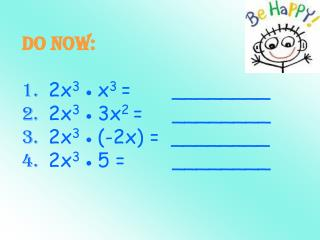DownloadDownload PresentationsO… 2x 3 (x 3 + 3x 2 - 2x + 5) =

sO… 2x 3 (x 3 + 3x 2 - 2x + 5) =

Télécharger la présentationsO… 2x 3 (x 3 + 3x 2 - 2x + 5) =

- - - - - - - - - - - - - - - - - - - - - - - - - - - E N D - - - - - - - - - - - - - - - - - - - - - - - - - - -
Presentation Transcript

1. Do Now:1. 2x3 x3 = ________2. 2x3 3x2 = ________3. 2x3 (-2x) = ________4. 2x3 5 = ________

2. sO…2x3(x3 + 3x2 - 2x + 5) =

3. The FOIL method is ONLY used when you multiply 2 binomials. It is an acronym and tells you which terms to multiply. Use the FOIL method to multiply the following binomials:(y + 3)(y + 7).

4. (y + 3)(y + 7). F tells you to multiply the FIRST terms of each binomial. y2

5. (y + 3)(y + 7). O tells you to multiply the OUTER terms of each binomial. y2+7y

6. (y + 3)(y + 7). I tells you to multiply the INNER terms of each binomial. y2 + 7y +3y

7. (y + 3)(y + 7). L tells you to multiply the LAST terms of each binomial. y2 + 7y + 3y + 21 Combine like terms. y2 + 10y + 21

8. Remember, FOIL reminds you to multiply the: First terms Outer terms Inner terms Last terms

9. The 2nd method is the Box Method. This method works for every problem! Here’s how you do it: (3x – 5)(5x + 2) Draw a box. Write a polynomial on the top & side of a box. It does not matter where. This will be modeled in the next problem along with FOIL.

10. 3) Multiply (3x - 5)(5x + 2) 15x2 First terms: Outer terms: Inner terms: Last terms: Combine like terms. 15x2 - 19x – 10 +6x -25x 15x2 -25x -10 +6x -10 You have 2 techniques. Pick the one you like the best!

11. First terms: Outer terms: Inner terms: Last terms: Combine like terms. 21p2 – 34p + 8 4) Multiply (7p - 2)(3p - 4) 21p2 -28p -6p 21p2 -6p +8 -28p +8

12. Multiply (y + 4)(y – 3) • y2 + y – 12 • y2 – y – 12 • y2 + 7y – 12 • y2 – 7y – 12 • y2 + y + 12 • y2 – y + 12 • y2 + 7y + 12 • y2 – 7y + 12

13. Multiply (2a – 3b)(2a + 4b) • 4a2 + 14ab – 12b2 • 4a2 – 14ab – 12b2 • 4a2 + 8ab – 6ba – 12b2 • 4a2 + 2ab – 12b2 • 4a2 – 2ab – 12b2

14. So, what if you have a binomial x trinomial? Please copy: (x + 3)(x² + 2x + 4)

15. Horizontal Method: (x + 3)(x² + 2x + 4)  = x³ + 2x² + 4x + 3x² + 6x + 12Write terms in descending order…  = x³ + 2x² + 3x² + 4x + 6x + 12 Combine like terms = x³ + 5x² + 10x + 12

16. Vertical Method: x² + 2x + 4 x + 3 x³ + 2x² + 4x 3x² + 6x + 12 x³ + 5x² + 10x + 12

17. OK, your turn… (x+1)(x²+2x+1) (b2 + 6b –7)(3b – 4) (2x2 + 5x –1)(4x – 3) (2y – 3)(3y2 – y + 5) (x2 + 2x + 1)(x + 2)